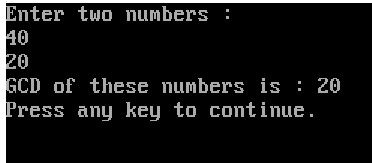+1 vote
295 views
Write a program in C++ to find the Greatest Common Divisor (G.C.D.) of two natural numbers. [March 2006]

Maharashtra board
| 295 views

+1 vote//Program to find the G.C.D. of two numbers

#include<iostream.h>
#include<conio.h>
int gcd(int a, int b);
void main()
{
int x, y, g;
clrscr();
cout<<"Enter two numbers : "<<endl;
cin>>x>>y;
g=gcd(x,y);
cout<<"GCD of these numbers is : "<<g;
getch();
}

int gcd(int a, int b)
{
int r;
r=a%b;
while (r>0)
{
a=b;
b=r;
r=a%b;
}
return b;
}

getch for more older version of Turbo C++ compiler

Enter two numbers :
40
20
GCD of these numbers is : 20
by Expert (10.7k points)